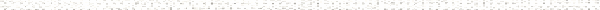A Precalculus Outline

 Chapter 8:  Systems of Equations and MatricesObjective: Systems of Equations and MatricesObjective: Understanding what it means for a point to be a solution to a system of linear equationsObjective: Classifying a system of linear equations as consistent or inconsistentObjective: Classifying a system of linear equations is dependent or independentObjective: Solving a system of linear equations by graphingObjective: Solving a system of linear equations using substitutionObjective: Solving a consistent independent system of linear equations by eliminationObjective: Solving a consistent dependent system of linear equations by eliminationObjective: Solving a system of linear equation by elimination and an applicationObjective: Solving "mixture" problemsObjective: Graphing linesObjective: Plotting points in the planeObjective: X-interceptsObjective: Y-interceptsObjective: Solving linear equationsObjective: Solving a system of linear equations using an augmented matrixObjective: Systems of Linear Equations: Gaussian EliminationObjective: How to add, subtract, and multiply a matrix by a real numberObjective: How to multiply two matrices togetherObjective: Properties of real number arithmetic Section 8.4: Systems of Linear Equations:  Matrix InversesObjective: Finding the inverse of a matrix using Gauss-Jordan EliminationObjective: Solving a system of linear equations using a matrix inverseObjective: Systems of Linear Equations: Gaussian EliminationObjective: Systems of Linear Equations: Augmented MatricesObjective: Matrix ArithmeticObjective: Determinants and Cramer's RuleObjective: Finding the determinant of a 2 x 2 matrixObjective: Finding the determinant of a 3 x 3 matrixObjective: Finding the determinant of a 4 x 4 matrixObjective: Solving a system of linear equations using Cramer's RuleObjective: Finding the inverse of a matrix using determinants (co-factors)Objective: Systems of Linear Equations: Gaussian EliminationObjective: Systems of Linear Equations: Matrix InversesObjective: Finding a partial fraction decomposition of a rational expression with distinct linear factorsObjective: Finding a partial fraction decomposition of a rational expression with repeated linear factorsObjective: Finding a partial fraction decomposition of a rational expression with an irreducible quadratic factorObjective: Finding a partial fraction decomposition of a rational expression where long division is required firstObjective: Overview of factoring polynomialsObjective: Factoring out greatest common factor part 1Objective: Factoring out greatest common factor part 2Objective: Factoring out greatest common factor part 3Objective: Factoring out greatest common factor part 4Objective: Difference of squares part 1Objective: Difference of squares part 2Objective: Difference of squares part 3Objective: Factoring trinomials part 1Objective: Factoring trinomials part 2Objective: Factoring trinomials part 3Objective: Factoring trinomials part 4Objective: Factoring trinomials part 5Objective: Factoring trinomialsObjective: How to perform polynomial long divisionObjective: Solving a system of non-linear equations graphicallyObjective: Solving a system of non-linear equations using substitutionObjective: Systems of Linear Equations: Gaussian EliminationObjective: Linear FunctionsObjective: Quadratic FunctionsObjective: CirclesObjective: ParabolasObjective: EllipsesObjective: Hyperbolas